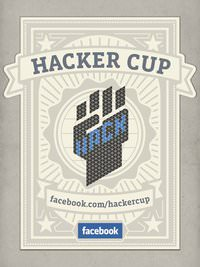# Facebook Hacker Cup – Double Squares.Facebook Hacker Cup 中文翻譯為 臉書駭客盃，雖然叫做駭客盃，實際上則是程式設計競賽。應該是屬於國際級的競賽吧? 這也是我第一次參加競賽以及第一次參與這類如此不同的競賽。而，這整個比賽是在線上進行，時間以格林威治標準時間為準，所以各地都有一定的時差存在。不過也剛好反映出FB的時間計算上的問題…

### Facebook Hacker Cup Qualification Round

Double Squares

A double-square number is an integer X which can be expressed as the sum of two perfect squares. For example, 10 is a double-square because 10 = 3² + 1². Your task in this problem is, given X, determine the number of ways in which it can be written as the sum of two squares. For example, 10 can only be written as 3² + 1² (we don’t count 1² + 3² as being different). On the other hand, 25 can be written as 5² + 0²or as 4² + 3².

Input

You should first read an integer N, the number of test cases. The next N lines will contain N values of X.
Constraints
0 ≤ X ≤ 2147483647
1 ≤ N ≤ 100

Output

For each value of X, you should output the number of ways to write X as the sum of two squares.

Example input

5
10
25
3
0
1

Example output

1
2
0
1
1

「為什麼要除以1000?」我問。

「你看喔，」他指著一長排由電腦輸出的結果，說：「這些結果根本不會超過它本身除以1000對吧? 所以我們如果加入這樣的判斷，那應該會快很多才對。」

「有道理，我們來試試看！」